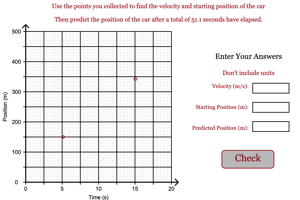Programs for Programs for Graphing Motion
Below are all the programs that might be used with this topic. Click on "See Resources" to see if there are any student directions, lab sheets or other materials that have been created for this program.
Graphing Motion Simple

Lab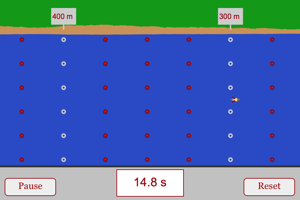Graphing Two Stage Motion

LabFreestyle Graphing of Motion

Lab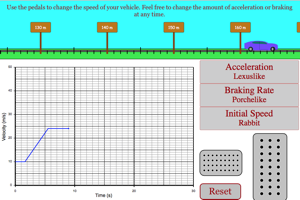Constant Velocity Graph

Homework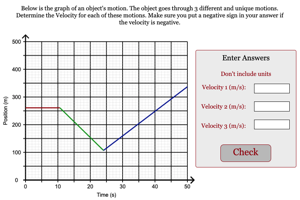Velocity Graph Matching Challenge

Game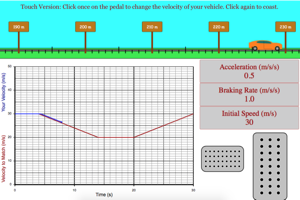Predictions from Constant Velocity Graph

HomeworkPredictions From Two Points of Motion

Homework